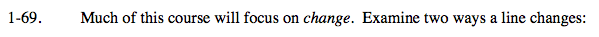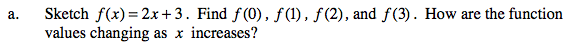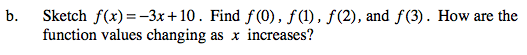### Home > CALC > Chapter 1 > Lesson 1.2.4 > Problem1-69

1-69.
1. Much of this course will focus on change. Examine two ways a line changes: Homework Help ✎

1. Sketch f(x) = 2x + 3. Find f(0), f(1), f(2), and f(3). How are the function values changing as x increases?

2. Sketch f(x) = −3x + 10. Find f(0), f(l), f(2), and f(3). How are the function values changing as x increases?How do the f(x) values change as x increases? Do these values increase, decrease or stay constant?How do the f(x) values change as x increases? Do these values increase, decrease or stay constant?

Use the eTool below to makes changes to the x values.
Click the link at right for the full version of the eTool: Calc 1-69 HW eTool